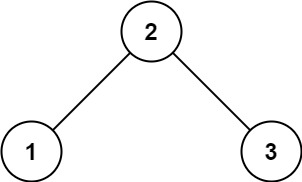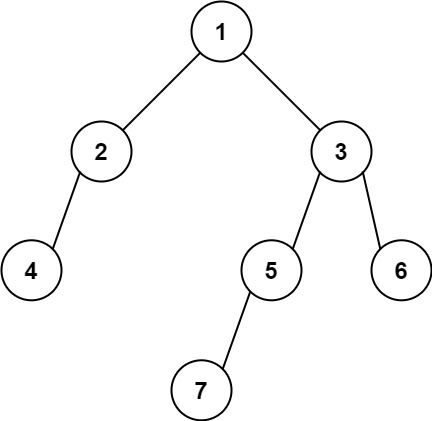# Find Bottom Left Tree Value

## Find Bottom Left Tree Value

Given the `root` of a binary tree, return the leftmost value in the last row of the tree.

Example 1:```Input: root = [2,1,3]
Output: 1
```

Example 2:```Input: root = [1,2,3,4,null,5,6,null,null,7]
Output: 7
```

Constraints:

• The number of nodes in the tree is in the range `[1, 104]`.
• `-231 <= Node.val <= 231 - 1`
Solution to the Above Question
/**
* Definition for a binary tree node.
* struct TreeNode {
*     int val;
*     TreeNode *left;
*     TreeNode *right;
*     TreeNode() : val(0), left(nullptr), right(nullptr) {}
*     TreeNode(int x) : val(x), left(nullptr), right(nullptr) {}
*     TreeNode(int x, TreeNode *left, TreeNode *right) : val(x), left(left), right(right) {}
* };
*/
class Solution {
public:
int findBottomLeftValue(TreeNode* root) {
vector<int>temp;
vector<vector<int>>v;
if(root==NULL){
return 0;
}
queue<TreeNode*>q;
q.push(root);
q.push(NULL);
while(!q.empty()){
TreeNode* f=q.front();
q.pop();
if(f==NULL){
v.push_back(temp);
temp.clear();
if(!q.empty()){
q.push(NULL);
}
}
else{
temp.push_back(f->val);
if(f->left){
q.push(f->left);
}
if(f->right){
q.push(f->right);
}
}
}
int k=v.size();